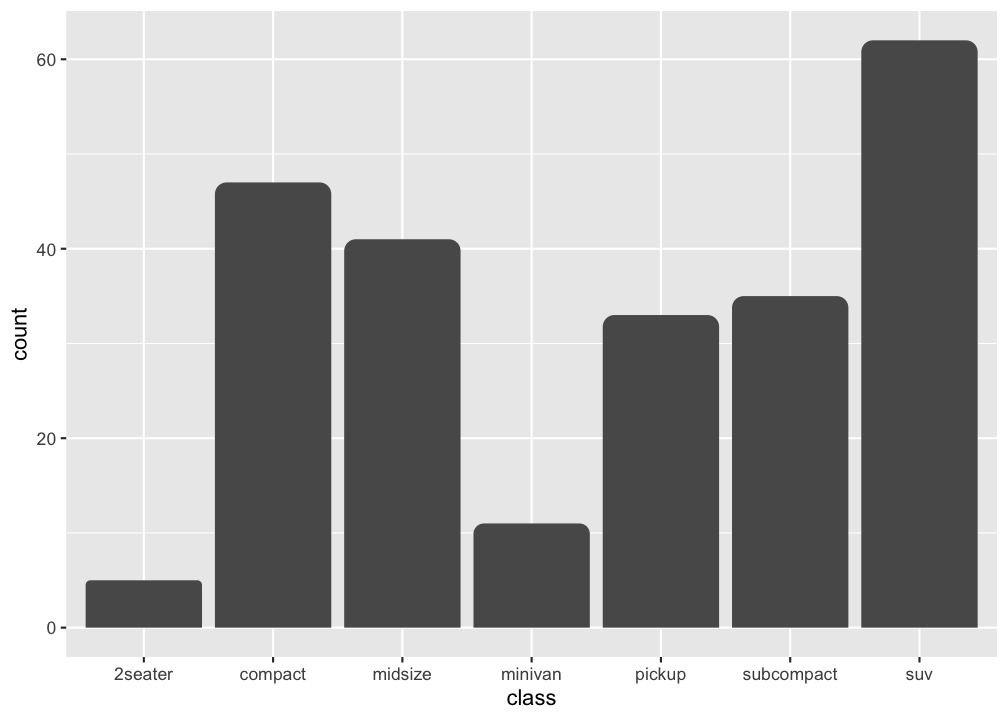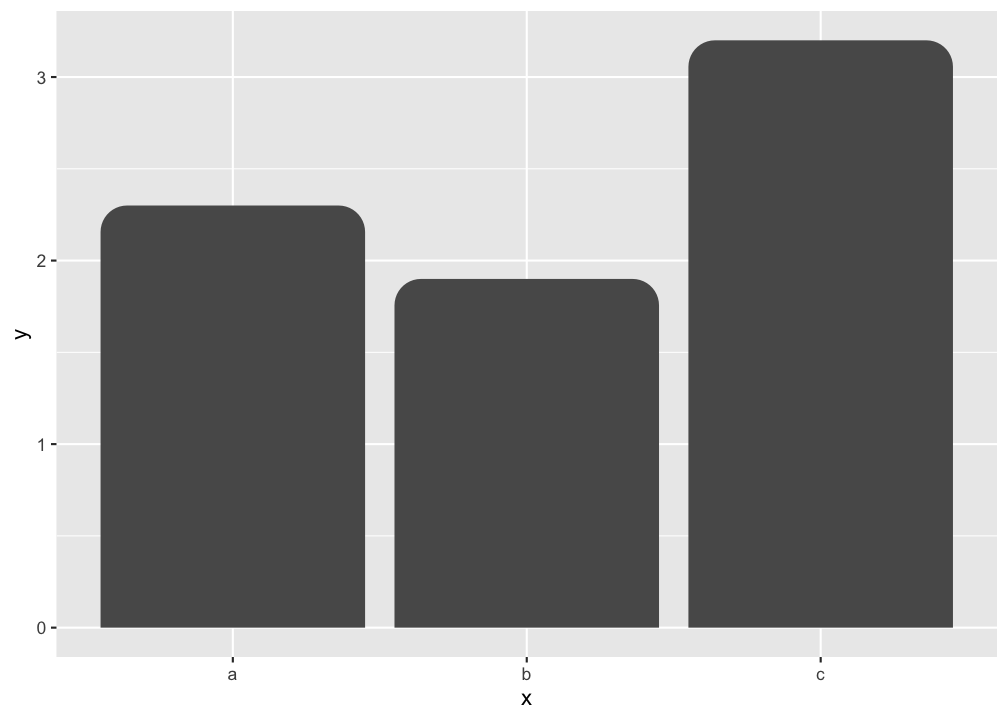ggrounded creates bar plots with rounded corners using ggplot2.

## Installation

Install the released version of ggrounded from CRAN:

install.packages("ggrounded")

Or install the development version from GitHub with:

# install.packages("pak")
pak::pak("botan/ggrounded")

## Usage

There are two types of bar charts in ggplot2: geom_bar() and geom_col(). geom_bar_rounded() and geom_col_rounded() are wrappers on them for rounding the top corners. geom_bar_rounded() makes the height of the bar proportional to the number of cases in each group (or if the weight aesthetic is supplied, the sum of the weights).

library(ggrounded)
library(ggplot2)

ggplot(mpg, aes(class)) +
geom_bar_rounded()If you want the heights of the bars to represent values in the data, use geom_col_rounded() instead.

ggplot(data.frame(x = letters[1:3], y = c(2.3, 1.9, 3.2)), aes(x, y)) +
geom_col_rounded()## Code of Conduct

Please note that the ggrounded is released with a contributor code of conduct. By contributing in this project you agree to abide by its terms.